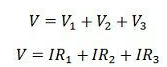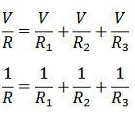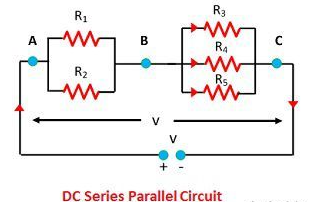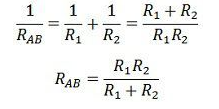# DC circuit And Its Types﻿DC Circuit -﻿The closed path in which the direct current flows is called the DC circuit. The current flows in only one direction and it is mostly used in low voltage applications. The resistor is the main component of the DC circuit.

A simple DC circuit is shown in the figure below which contains a DC source (battery), a load lamp, a switch, connecting leads, and measuring instruments like ammeter and voltmeter. The load resistor is connected in series, parallel or series-parallel combination as per requirement.﻿Types Of DC Circuit-﻿DC Circuit is mainly classified into 3 types:

1. series DC Circuit
2. Parallel DC Circuit
3. Series and Parallel DC Circuit

﻿Series DC Circuit

﻿When all the resistive components of a DC circuit are connected end to end to form a single path for flowing current, then the circuit is referred to as a series DC circuit. The manner of connecting components end to end is known as a series connection.

The figure below shows the simple series circuit. In the series circuit the resistor R1, R2, and R3 are connected in series across a supply voltage of V volts. The same current I is flowing through all the three resistors.﻿If V1, V2, and V3 are the voltage drop across the three resistor R1, R2, and R3 respectively, thenLet R be the total resistance of the circuit then,Total resistance = Sum of the individual resistance.

In such type of circuit all the lamps are controlled by the single switch and they cannot be controlled individually. The most common application of this circuit is for decoration purpose where a number of low voltage lamps are connected in series.

﻿Parallel DC Circuit

When two or more electrical components are connected in a way that one end of each component is connected to a common point and the other end is connected to another common point, then the electrical components are said to be connected in parallel, and such an electrical DC circuit is referred as a parallel DC circuit.

The figure shows a simple parallel circuit. In this circuit the three resistor R1, R2, and R3 are connected in parallel across a supply voltage of V volts. The current flowing through them is I1, I2 and I3 respectively.The total current drawn by the circuit isLet R be the total or effective resistance of the circuit, thenReciprocal of total resistance = sum of reciprocal of the individual resistance..

All the resistance is operated to the same voltage, therefore all of them are connected in parallel. Each of them can be controlled individually with the help of a separate switch.

﻿Series-Parallel DC Circuit

﻿The circuit in which series and parallel circuit are connected in series is called a series parallel circuit. The figure below show the series-parallel circuit. In this circuit, two resistor R1 and R2 are connected in parallel with each other across terminal AB. The other three resistors R3, R4 and R6 are connected in parallel with each other across terminal BC.The two groups of resistor RAB and RBC are connected in series with each other across the supply voltage of V volts. The total or effective resistance of the whole circuit can be determined as given below:Similarly,Total or effective resistance of the circuit,

R=RAB+RBC